HOTS & Value Based Questions: Introduction to Trigonometry

# HOTS & Value Based Questions: Introduction to Trigonometry - Notes | Study Mathematics (Maths) Class 10 - Class 10

 Table of contentsHOTSValue-based Questions1 Crore+ students have signed up on EduRev. Have you?

HOTS

Q1. What is the maximum value of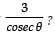Hint: ∵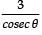= 3 sin θ
Since the maximum value of ‘sin θ’ is 1
∴  the maximum value of 3 sin θ is 3 × 1 i.e. 3

⇒  the maximum value ofis 3.

Q2. If sin θ = 1/3,  then find the value of 9 cot2θ + 9.

Hint: cot2 θ = cosec2 θ – 1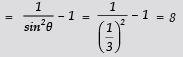∴ 9 cot2θ + 9 = 9(8) + 9 = 72 + 9 = 81

Q3. If 4 tan θ = 3, then find the value of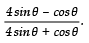Hint: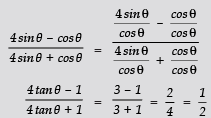Q4. If sin α = 1/2 and cos β = 1/2 then find the value of (α + β).

Hint: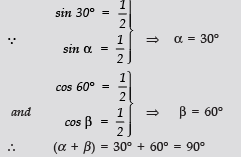Q5. If sin θ + cos θ = √3 , find the value of tan θ + cot θ .

Hint: sin θ + cos θ = 3 ⇒ (sin θ + cos θ)2 = 3
⇒ sin2 θ + cos2 θ + 2 sin θ . cos θ = 3
⇒ 1 + 2 sin θ . cos θ = 3 ⇒ 2 sin θ . cos θ = 2    [∴ sin2θ + cos2θ = 1]

⇒ sin θ . cos θ =1 ⇒  1 =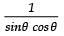⇒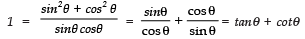Thus, tanθ + cotθ = 1

Q6. cos (A+B) = 1/2 and sin (A–B) = 1/2 ; 0° < (A + B) < 90° and (A – B) > 0°. What are the values of ∠A and ∠B?

Hint: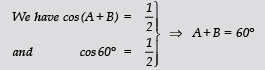...(1)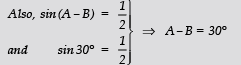...(2)

Adding (1) and (2), 2A = 90 ⇒ A = 45
From (1) 45° + B = 60°  ⇒   B = 60° – 45° = 15°
Thus, ∠A = 45° and ∠B = 15°

Value-based Questions

Q1. A group of students plan to put up a banner in favour of respect towards girls and women, against a wall. They placed a ladder against the wall such that its foot is at a distance of 2.5 m from the wall and its top reaches a window 6 m above the ground.

(i) Find the length of the ladder.
(ii) Which mathematical concept is used in this problem?
(iii) By putting up a banner in favour of respect to women, which value is depicted by the group of students?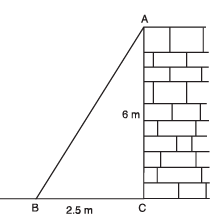Sol. (i) Let AB be the ladder and CA be the wall with the window at A. Also,
BC = 2.5 m
CA = 6m
∴ In rt ΔACB, we have
AB2 = BC2 + CA2 [Using Pythagoras Theorem]
= (2.5)2 + (6)2
= 6.25 + 36 = 42.25

⇒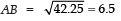Thus, the length of the ladder is 6.5 m.
(ii) Triangles (Pythagoras Theorem)
(iii) Creating positive awareness in public towards regards to women.

The document HOTS & Value Based Questions: Introduction to Trigonometry - Notes | Study Mathematics (Maths) Class 10 - Class 10 is a part of the Class 10 Course Mathematics (Maths) Class 10.
All you need of Class 10 at this link: Class 10

## Mathematics (Maths) Class 10

53 videos|403 docs|138 tests
 Use Code STAYHOME200 and get INR 200 additional OFF

## Mathematics (Maths) Class 10

53 videos|403 docs|138 tests

Track your progress, build streaks, highlight & save important lessons and more!

,

,

,

,

,

,

,

,

,

,

,

,

,

,

,

,

,

,

,

,

,

;